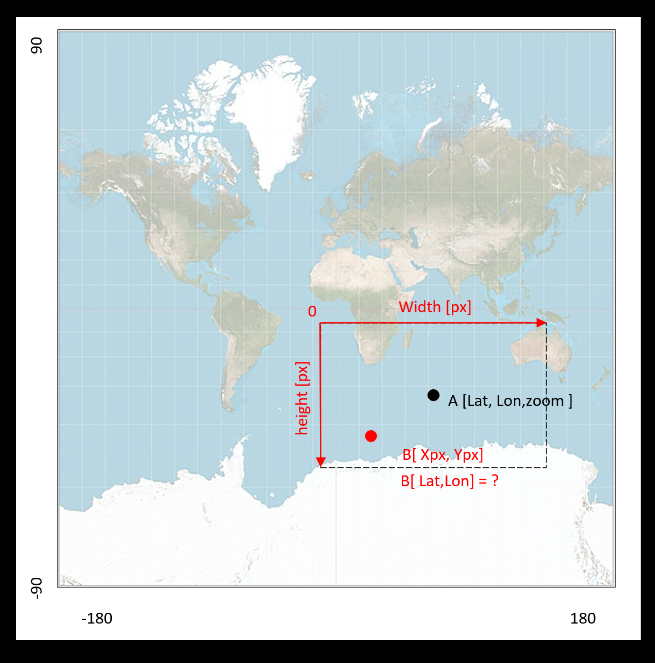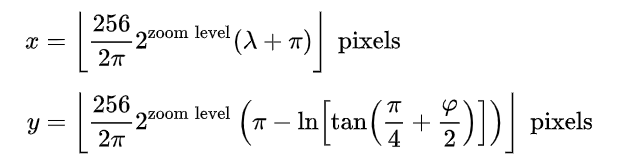# Transform x,y pixel values into lat and long

 0 Hi I have a little problem here. I have latitude, longitude and zoom of center of my map window (openstreet map). I have dimension map window. I also have pixel positions of specific events on the map in the window. Can Anyone help me how to convert these pixel positions into latitude and longitude coordinates or any other coordinate system that I will be able to visualize on different map later ? Here is an illustration of my problem.asked 09 Jul '20, 08:24Jozef 11●1●1●2 accept rate: 0%

 0 The projection you are displaying the map in is the so called spherical Mercator projection, you can convert Mercator coordinates to WGS84 lat/lon with the formulas from https://wiki.openstreetmap.org/wiki/Mercator (you will need to scale from you screen pixels or whatever to Mercator meters but that is a simple exercise left for the reader). Further note: Mercator latitude coordinates do not actually cover -90° to 90° degrees as your image would suggest. answered 09 Jul '20, 12:19SimonPoole ♦ 44.6k●13●324●700 accept rate: 18%
 0 Thank I have found the solution using this formula (https://en.wikipedia.org/wiki/Web_Mercator_projection )calculate the x,y position of my center point transform coordinate system of window to global inverse transformation code below ``````def LatLontoXY(lat_center,lon_center,zoom): C =(256/(2*pi) )* 2**zoom x=C*(math.radians(lon_center)+pi) y=C*(pi-math.log( math.tan( (pi/4) + math.radians(lat_center)/2 ) )) return x,y def xy2LatLon(lat_center,lon_center,zoom,width_internal,height_internal,pxX_internal,pxY_internal): xcenter,ycenter=LatLontoXY(lat_center,lon_center,zoom) xPoint=xcenter- (width_internal/2-pxX_internal) ypoint=ycenter -(height_internal/2-pxY_internal) C = (256 / (2 * pi)) * 2 ** zoom M = (xPoint/C)-pi N =-(ypoint/C) + pi lon_Point =math.degrees(M) lat_Point =math.degrees( (math.atan( math.e**N)-(pi/4))*2 ) return lat_Point,lon_Point `````` answered 09 Jul '20, 15:43Jozef 11●1●1●2 accept rate: 0%
 toggle preview community wiki:

By Email:

Markdown Basics

• *italic* or _italic_
• **bold** or __bold__
• image?![alt text](/path/img.jpg "title")
• numbered list: 1. Foo 2. Bar
• to add a line break simply add two spaces to where you would like the new line to be.
• basic HTML tags are also supported

Question tags:

×529
×77
×2

question asked: 09 Jul '20, 08:24

question was seen: 6,334 times

last updated: 09 Jul '20, 15:43